In Section 3.2.1 of _[Seven Sketches](http://math.mit.edu/~dspivak/teaching/sp18/7Sketches.pdf)_ - and please download today's new version of the book! - Brendan Fong and David Spivak describe a nice way to get categories from graphs. It's very simple, yet important for building databases. Here's the basic idea. Start with a graph, for example this: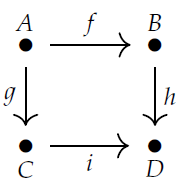It has a bunch of "edges", like \$$f,g,h,i\$$, going between "nodes" like \$$A,B,C,D\$$. Then build a category where the objects are the nodes and the morphisms are the paths of edges. We compose morphisms by attaching one path onto the end of another. For example \$$f\$$ is a path of length 1 from \$$A\$$ to \$$B\$$, so it gives a morphism \$$f: A \to B \$$. \$$h\$$ is a path of length 1 from \$$B\$$ to \$$D\$$, so it gives a morphism \$$g: B \to D\$$. We can compose these two morphisms and get \$$h \circ f : A \to D\$$, which is a path of length 2 from \$$A\$$ to \$$D\$$. There's also another morphism from \$$A\$$ to \$$D\$$, namely \$$i \circ g : A \to D\$$. In this example the longest paths have length 2, but in general a path could have any natural number as its length, including 0. Paths of length 0 are important because they give the identity morphisms in our category. For example, there's a path of length 0 from \$$A\$$ to \$$A\$$, which you can't see because it has no edges! It gives the identity morphisms \$$1_A: A \to A\$$. When we build a category from a graph this way, it's called the **free category** on that graph. And if we call the graph \$$G\$$, we call the free category on that graph \$$\mathbf{Free}(G)\$$. I should warn you that different people mean different things by "graph", depending on: * whether we put arrows on the edges, * whether we allow more than one edge going from one node to another, * whether we allow edges going from a node to itself, * whether the edges have names, and * whether we allow infinitely many nodes and edges. But category theorists have a very specific thing in mind when we say "graph". We are very generous: we say _yes_ to all these questions! So, for example, we allow this graph: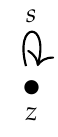This graph has just one edge. But the free category on this graph has infinitely many morphisms, namely $1_z : z \to z$ $s : z \to z$ $s \circ s : z \to z$ $s \circ s \circ s : z \to z$ and so on. There's one morphism for each natural number. In fact, this is how category theorists often think about the natural numbers! We also allow this graph: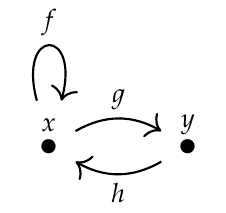**Puzzle 104.** How many paths of length \$$n\$$ go from \$$x\$$ to \$$x\$$ in this graph? The answer is a famous sequence of numbers. So, you're getting a famous sequence from the free category on a graph! It may sound complicated to let our graphs have so many features, but it's actually more complicated to _disallow_ them. The category theorists' definition of graph is very simple: **Definition.** A **graph** \$$G\$$ is a set \$$N\$$ of **nodes**, a set \$$E\$$ of **edges**, a function \$$s : E \to N\$$ assigning each edge its **source**, and a function \$$t: E \to N\$$ assigning each edge its **target**. You can visualize the source and target of an edge using this picture: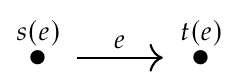but remember: it's possible to have \$$s(e) = t(e)\$$. If you're a stickler for detail, you may also want to see the precise definition of a "path": **Definition.** Given a graph, a **path** from a node \$$x\$$ to a node \$$y\$$ is a finite sequence of edges \$$(e_1, \dots, e_n)\$$ with $s(e_1) = x, \quad t(e_1) = s(e_2), \quad \dots, \quad t(e_{n-1}) = s(e_n), \quad t(e_n) = y.$ Here \$$n = 0, 1, 2, 3, \dots\$$ is called the **length** of the path. Okay, I think that's enough for today! Next time we'll use this idea to think about databases. For now you should try these exercises, or at least look at other students' solutions: **[Exercise 3](https://forum.azimuthproject.org/discussion/2131/p1).** Show that \$$\textbf{Free}(G)\$$ really is a category. **[Exercise 4](https://forum.azimuthproject.org/discussion/2132/p1).** Completely work out the set of morphisms between each pair of objects in the category called \$$\textbf{3}\$$, which is $\textbf{Free}( [ v_1 \overset{f_1}{\rightarrow} v_2 \overset{f_2}{\rightarrow} v_3 ] ) .$ **[Exercise 5](https://forum.azimuthproject.org/discussion/2133/p1).** The category \$$\textbf{3}\$$ is an example of a general idea. For each natural number \$$n\$$ there's a category \$$\mathbf{n}\$$ that works the same way. Figure out what it is, and figure out the total number of morphisms in this category! And here's a puzzle for people who love numbers. I'll admit it: I love numbers.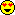But math is not only about numbers, so if you don't love them, don't worry: this puzzle is not important for this course, though it _is_ part of a big subject in math: **Puzzle 105**. It's been known since at least the fifth century BC that $\sqrt 2 = 1 + \cfrac{1}{2 + \cfrac{1}{2 + \cfrac{1}{2 + \cfrac{1}{2 + \cfrac{1}{2 + \ddots\,}}}}}$ If we cut off this continued fraction we get these rational approximations to \$$\sqrt{2}\$$: $\frac11, \frac32, \frac75, \frac{17}{12}, \frac{41}{29}, \frac{99}{70}, \dots$ where the denominator of the \$$n\$$th fraction in this list is called the **[Pell number](https://en.wikipedia.org/wiki/Pell_number)** \$$P_n\$$ and the numerator is \$$P_n + P_{n-1}\$$. Find a graph with two nodes \$$x\$$ and \$$y\$$ such that the number of paths of length \$$n\$$ from \$$x\$$ to \$$y\$$ is the \$$n\$$th Pell number. **[To read other lectures go here.](http://www.azimuthproject.org/azimuth/show/Applied+Category+Theory#Chapter_3)**